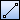# Creating Line

The following 4 methods can be used to create a line.

• Specifying the "start point" and "end point" of a line.
• Specifying the "start point," "length" and "end point" of a line.
• Specifying the "start point," "angle" and "end point" of a line.
• Specifying the "start point," "length," "angle" and "end point" of a line.
1. Point [Line] on [Shape] menu, and click [Line].
Then you will be able to create a line.
2.  Supplemental ▪ You can enter into the line creating status by clickingon [Shape] toolbar.

### When specifying the "start point" and "end point" of a line

1. Click the start point of a line on the drawing.
2. Click the end point of the line on the drawing.
A line to connect the start and end points will be created.

### When specifying the "start point," "length" and "end point" of a line

1. Click the start point of a line on the drawing.
2. Input a distance from the start point in [(Length)] box on Command Window.
3. Click the end point of the line on the drawing.
A line with a specified length will be created toward the end point direction.

### When specifying the "start point," "angle" and "end point" of a line

1. Click the start point of a line on the drawing.
2. Input an angle for the line in [(Angle)] box on Command Window.
3. Click the end point of the line on the drawing.
A line with a specified angle will be created toward the end point direction.

### When specifying the "start point," "length," "angle" and "end point" of a line

1. Click the start point of a line on the drawing.
2. Input a distance from the start point in [(Length)] box on Command Window.
3. Input an angle for the line in [(Angle)] box on Command Window.
4. Click the end point of the line on the drawing.
A line with a specified length and angle will be created toward the end point direction.
 Supplemental ▪ You can specify the start point, end point and through point by inputting X and Y coordinates in [Start Point], [End Point] and [Through Point] boxes on Command Window. ▪ To input a relative position from the start point as the end point, input coordinate values using [@X coordinate , (comma) Y coordinate] format in [End Point] box on Command Window.